## NUMEROLOGY

Numerologists assign different percentages of which they call Core Energy to each person. The birth-date, which constitutes 40% of your Core Energy, is how your Life-Path is derived. The Birthday, which constitutes 10%, is obviously the number of your birthday, reduced to a single digit. For instance if your birthday is the 23rd, your Birthday would be 5, adding the two digits 2+3=5 to obtain a single digit. The Expression, which constitutes 30% of the Core Energy, is derived from your full name. For the purpose of these codes it is the name you are using today, not necessarily the name on your birth certificate. The vowels of the name, which constitute 20% of the Core Energy, are how we determine your Soul Urge.

In a nutshell, connecting your Core Energy numbers to the numbers of the 22 cards or major arcana trumps, is one of the major means we discover your hidden codes. However, because Numerology only uses the numbers one to nine, and the two master numbers 11 and 22, this only involves the Tarot trumps or cards: The Magician, 1; The High Priestess, 2; The Empress 3; The Emperor 4; The Hierophant 5; The Lovers 6; The Chariot 7; Strength 8; The Hermit 9 and Justice 11. Two trumps or cards are used for the master number 22, The Fool 0 and The World 21 as both cards are thought to represent the energies of zero and twenty-one.

To give you an example, to determine the Life-Path and Birthday, of the birth-date of August 3rd, 1956, in numerology this date would be written 8+3+3. The first and second numbers are self-explanatory, being the number of the month and day of your Birth-date.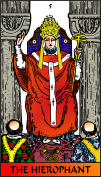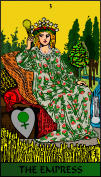The year is always reduced to either a number between 1 and 9 or one of the master numbers 11 and 22. For instance, 1956 reduces to 3 thus: 1+9+5+6=21. Then 21 is further reduced to a single digit by adding 2+1=3. In conclusion 8+3+3=14, which when added together 1+4=5 produces a Life-Path of 5, which equates to card/trump number 5, The Hierophant. The Birthday, which in this case is 3, equates to card/trump number 3, The Empress.

To understand the other two Core Energy elements, the Expression and the Soul Urge lets take the name John Brown. First each letter is assigned a number between 1 and 9: J=1 O=6 H=8 N=5 B=2 R=9 O=6 W=5 N=5. Below is the traditional way numerologists determine the value of each letter.

ENGLISH ALPHABET ASSIGNED NUMBER VALUES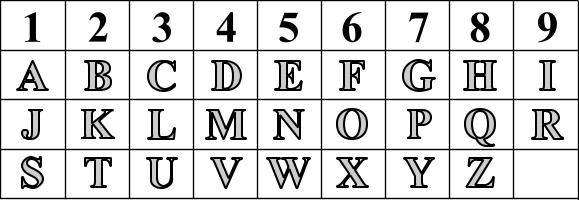Figure 1. Table of letter values according to Numerology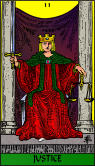Once a value for each letter is established, then the values are added together, which results in a total of 47. This number is then reduced further 4+7 = 11. Making the Expression the master number 11, which equates to the card/trump number 11, Justice. This same process is used to obtain the Soul Urge, using only the vowels of the name. By means of this formula the Soul Urge will be determined to be 3, O=6 + O=6 = 12 1+2=3, which again equates to card/trump number 3, the Empress.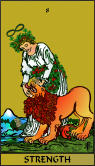Ordinarily, in traditional numerology these four elements are the only important factors. Nonetheless, because of the spiritual meaning of the Hebrew letters, which are assigned to the twenty-two cards or trumps of the Tarots Major Arcana, the consonants of the name are of vital importance in the codes. This is because the consonants of your name give you the Secret Self. John Browns Secret Self is determined using the same process as above, only as we said just the consonants; i.e. J=1 H=8 N=5 B=2 R=9 W=5 N=5 = 35. Again this number needs to be further reduced to a single digit 3+5=8. This equates to card/trump number 8, Strength.

The only other numbers relevant in traditional Numerology were concerned with what was referred to as karmic numbers. In traditional Numerology you were connected to a karmic number, if any or your Core Energy elements was reached through thirteen, fourteen, sixteen or nineteen. To recap, the traditional Core Energy elements are (Life-Path, (birth-date) Birthday, Expression (full name) and Soul Urge (vowels). However, as we said, for the purpose of the Codes, the Secret Self as a Core Energy element needs to be added. This is because although no percentage of Core Energy is assigned to the Secret Self, it is included in determining your total number. In addition, the number fifteen should be included as a karmic number.

Incidentally, having a karmic number traditionally meant that you were suffering in this life for past life misdeeds. However, because this is the fullness of time, anyone who was at least twenty-one by the Winter Solstice of 2004 chose to be born at this time not to absolve his or her bad karma, but to assist in the transformation of the world. Of course many will not remember in time to fulfill their purpose. But those who awaken in time can literally change the world. As we said that is the codes purpose to help you remember.There are multiple other numerological factors used in the codes, such as the Total Number as we said deduced by adding the Life-Path, Birthday, Expression, Soul-Urge and Secret Self. Using the above example these numbers will be 5+3+11+3+8 = 30. By further reducing the number, 3+0=3 we arrive at a Total Number of 3, which again equates to card/trump number 3, the Empress.

Back to List at Top of Page